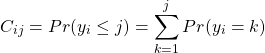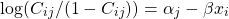# What is important for a loan?

Few months back I read this post, which referred to this amazing. The numbers are for individuals who borrowed money, amounts, term and conditions of the loans and much more. Most of the people naturally paid back the loan in full, however, some did not,

and from those unfortunates we can derive some insight. I kick out all the people that actually paid back the full loan, they form the bulk of the data and will not be useful for our purpose, which is to check what are the variables that make people more likely to be late with payment or to default altogether. We are left with 178 individuals who are in “Grace period”, “Late (16-30 days)” ,”Late (31-120 days)” ,”Performing Payment Plan” and “Default”. This suits nicely into an ordered logit model. Data has lots of characteristics available for these individuals, I picked some that dim reasonable and are interesting to look at. Do the loan rate affect the probability of paying it back? Does the duration of the loan matter? Does the amount you borrow? Level of income?

Discrete choice models deal with discrete dependent variable. Logit model for binary (zero or one..), multinomial model for larger number of choices, e.g. different brands. In our case we exploit the fact that we can order the dependent variable, that is, “Late (31-120 days)” is worse than “Late (16-30 days)”. In the ordered logit model the probability of y being less than j is the sum of probabilities of y being equal to all categories less than or equal to j. Same way the probability for roll of a fair dice being less than 2 is equal to the sum of it being equal to 1 and being equal to 2, more formally:Now we simply take the transform the dependent into the following:So the transformed dependent variable (log of the odds ratio..) is linear in the independent variables. This can be easily implemented using the function “polr” in R. As always code is below.
Take a look at the following figure, it is the coefficients from the ordered logit model.The model is non-linear, just google ‘ordered logit’ if you want to know more about it. For now, a coefficient means that the specific variable increase or decrease the probability of moving from one level to another. A large negative bar for the ‘interest rate’ variable (IR), means the higher the rate, the LESS likely the person to shift from “Late (16-30 days)” state to “Late (31-120 days)” state. Well, it is natural to have such an impact since the IR itself is determined according to how ‘risky’ is the individual. The more ‘risky’ she is, the more compensation (higher rate of return) is needed. We can see the amount you borrow, contrary to what I expected, has no major effect. On the other hand, ‘Debt to Income ratio’ has positive effect meaning the higher the ratio the more likely you are to shift into a worse category, which makes perfect sense. Taking a loan for longer periods also seem to worsen the situation, so better short than long, even though I am guessing it might be correlated with the “income to debt ratio”, since if you have higher debt with respect to your income you would like to spread the installments over longer period. Owning a house boosts the credibility of the borrower relatively to a borrower that rents or have a standing mortgage.

Code and references are below.

References: